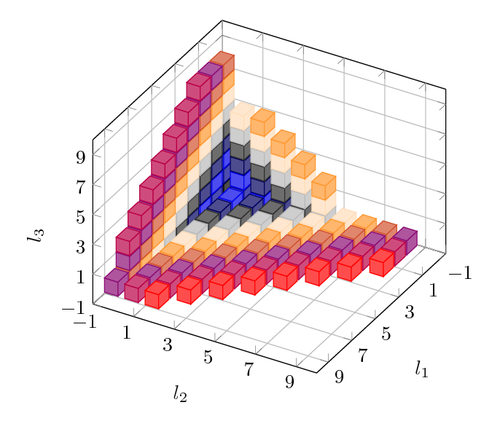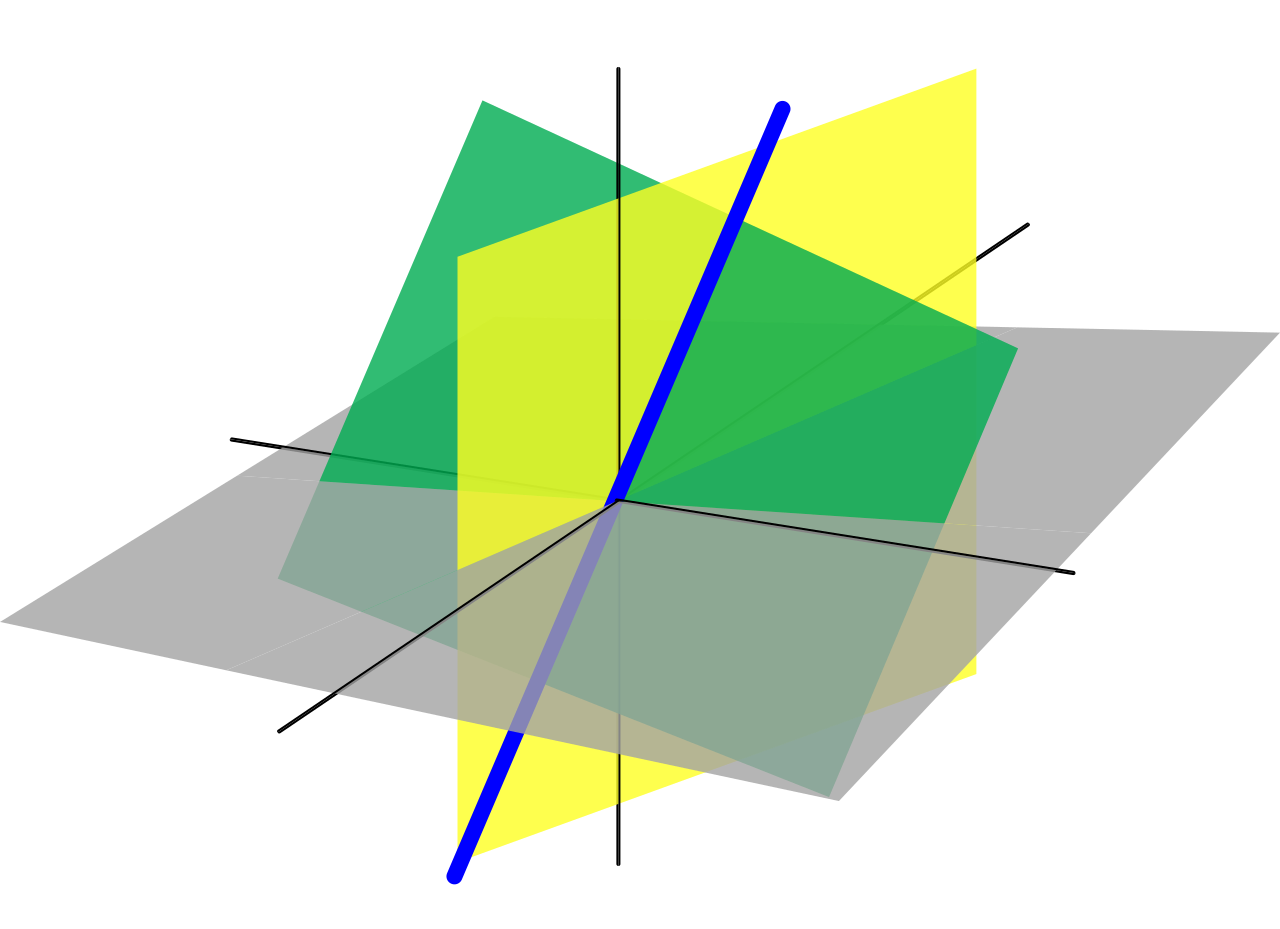Solution to 35C3 Junior CTF challenge flagsflags

web

500 (variable)

Writeup

This was the challenge we got:

Fun with flags: http://35.207.169.47

Flag is at /flag

Difficulty estimate: Easy

Walkthrough

We got the server side code, which looks like this:

<?php
highlight_file(__FILE__);
\$lang = explode(',', \$lang);
\$lang = str_replace('../', '', \$lang);
\$c = file_get_contents("flags/\$lang");
if (!\$c) \$c = file_get_contents("flags/ot");
echo '<img src="data:image/jpeg;base64,' . base64_encode(\$c) . '">');

The objective was to read the file at /flag on the file system. When loading the web page, one is most likely to get a warning from PHP hinting that there is no file at the flags/\$lang path from PHP, where \$lang is the user’s language.

The embedded PHP suggests that whatever is at that file path is Base64 encoded and pushed directly into the image part of the page response.

By manipulating the Accept-Language request header, one can alter what file is requested. But, all input values are sanitised by replacing "../" in the input string with "".

By changing the request header to

Accept-Language: ....//....//....//....//flag

<img src="data:image/jpeg;base64,MzVjM190aGlzX2ZsYWdfaXNfdGhlX2JlNXRfZmw0Zwo=">

which, when decoded, yields the flag 35c3\_this\_flag\_is\_the\_be5t\_fl4g.

Solution to 35C3 Junior CTF challenge DecryptedDecrypted

crypto

500 (variable)

Writeup

This challenge was a part of a group of 12 challenges that all shared the same large code base.

The function that we needed to crack in order to get the flag was this one:

@app.route("/wee/encryptiontest", methods=["GET"])
def encryptiontest():
global encrypted
if not encrypted:
wee = """
# we use weelang to encrypt secrets completely secret

record pubkey
n: string
e: string
end

var key = pubkey('951477056381671188036079180681828396446164466568923964269373812360568216940258578681673755725586138473475522188240856850626984093905399964041687626629414562063470963902807801143023140969
20823423927677839717181758259182700869005678976353417411986304610681351575086373354375831981119478424684513892149555631145818047853885655084250969268639667911790304014860764271083257383802727400495207251674916842
54346976900167073270029894070147537353137306531896615417508808552131659375645782924643791678577787591364741734258313403069197056729334867119393339537506377299674551184754083697516025382028181906639397068860930465
26104043062374288648189070207772477271879494000411582080352364098957455090381238978031676375437980396931371164061080967754225429135119036489128165414029872153856547376448552882344531325480944511714482341088742350
11009737276674836492694100044152415782485951155734267352438805604935836260092517229999071999887386803819455546500803649793294581284534063885339973272198722848685819397907391376176037076960934762279549898730682241
31342367496077356579676679029666679967972413646887939190664453605477491938458252983426262889901587301497273983541920536923607163838510512716185590750480128002352503878370525735411578459589488569540357589151578719
93646182544696043757263004887914724250286341123038686355398997399922927237477691269351791943572679717263938613148630387793458838416117454016370454288153779764863162055098229903413503857354581027436855574871814478
74723799961787902440740395490598696972133680325877451439760094717565024267419349661465226715875381735013630562026807645781307072609924868164261206320317044245340505145587752470936697306277403704477207972070374382
86953511989843348305321935645259169014617255384187145173023908500495438565426993913390759768430286540045521692775713390171616970133736227701154066810802949947906265571171298204579880459740095301856221139515408199
39983153190486345031549722007896699102268137425607039925174692583738394816628508716999668221820730737934785438568198334912127263127241407430459511422030656861043544813130287622862247904749760983465608684778389799
70377087793187526885852470299176745072077367763985697993040450875510062484434182989649790682452018005103877912656386045303903577945538773305634383377680271619413807252827814278690190434340737764900098814225536986
0324110311816186668720584468851089864315465497405748709976389375632079690963423708940060402561050963276766635011726613211018206198125893007608417148033891841809', '3')

fun encrypt(message: string, key: pubkey): string
return bigModPow(strToBig(message), key.e, key.n)
end

fun get_cypher(key: pubkey): string
var message = '{}'
return encrypt(message, key)
end

""".format(DECRYPTED)
encrypted = runwee(wee)
return jsonify({"enc": encrypted})

And calling that function gave us this ciphertext in form of a large number:

650802889626540392576254226480769958677174063746262298961949406725587937603370598056914641680440287141866554424868358513810586735136666559905873773370795301824775736764582520414393058823900835653671443326759384479590622850329114068561701339992264327486363426970702107667234446480134526246514585103292832378240690398119481568246551291749012927947948046185733533974179911092159848587

The encryption code is a simple school book example on how to implement RSA with a classical mistake of not using any padding.

The public key is also quite big, 2466 decimal digits, or 8192 bits. The encrypted flag is not that big however: 381 decimal digits, or 1266 bits. This makes the modulus part of RSA to not kick in, and the RSA algorithm is reduced from

y = x^e mod n

to

y = x^e

And this is trivially solved by taking the cube root of the number since the exponent, key.e, is 3.

We wrote this sage program to solve it:

from sage.crypto.util import bin_to_ascii, ascii_to_bin

a = Integer(650802889626540392576254226480769958677174063746262298961949406725587937603370598056914641680440287141866554424868358513810586735136666559905873773370795301824775736764582520414393058823900835653671443326759384479590622850329114068561701339992264327486363426970702107667234446480134526246514585103292832378240690398119481568246551291749012927947948046185733533974179911092159848587)
p = pow(a, 1/3)
pa = p.ceil().binary()
pb = "00" + pa;
print(bin_to_ascii(pb))

The flag was: 35C3_OUR_CRYPTO_IS_AS_LEGIT_AS_MOST_CRYPTO_CURRENCIES

Solution to 35C3 Junior CTF challenge DANCEdDANCEd

crypto

500 (variable)

Writeup

This was the challenge description:

Sign up now, for the dance class you always wanted to visit! Totally secure, totally awesome! But be quick, the first few spots are already taken!

nc 35.207.159.114 1337

Source

Difficulty estimate: Medium

And we also got the source for the server, written in Go, here.

The server logic is pretty straightforward. You log in to it using Telnet and can do two things that matter: either register yourself for a dance class, or list all the existing registrations which are encrypted.

Reading through the server code shows that for each encryption, a 64 byte “random” number is generated based on the number of previous registrations, 2 secret numbers and a lot of fixed values. That random number is then XORed to the combination of dance class and name. We guess that the flag is in the name of the first registrant.

To be able to decrypt the first registration, we first need to figure out the two secret numbers that are used to generate the 64 byte number. The generating function looks like this:

func doubleRound(block *uint32) {
for i := 0; i < 4; i++ {
block[(1+i)%4][i] = block[(1+i)%4][i] ^ bits.RotateLeft32(block[(0+i)%4][i]+block[(3+i)%4][i], 7)
block[(2+i)%4][i] = block[(2+i)%4][i] ^ bits.RotateLeft32(block[(1+i)%4][i]+block[(0+i)%4][i], 9)
block[(3+i)%4][i] = block[(3+i)%4][i] ^ bits.RotateLeft32(block[(2+i)%4][i]+block[(1+i)%4][i], 13)
block[(0+i)%4][i] = block[(0+i)%4][i] ^ bits.RotateLeft32(block[(3+i)%4][i]+block[(2+i)%4][i], 18)
}
for i := 0; i < 4; i++ {
block[i][(1+i)%4] = block[i][(1+i)%4] ^ bits.RotateLeft32(block[i][(0+i)%4]+block[i][(3+i)%4], 7)
block[i][(2+i)%4] = block[i][(2+i)%4] ^ bits.RotateLeft32(block[i][(1+i)%4]+block[i][(0+i)%4], 9)
block[i][(3+i)%4] = block[i][(3+i)%4] ^ bits.RotateLeft32(block[i][(2+i)%4]+block[i][(1+i)%4], 13)
block[i][(0+i)%4] = block[i][(0+i)%4] ^ bits.RotateLeft32(block[i][(3+i)%4]+block[i][(2+i)%4], 18)
}
}

func generateKeyStream(count uint32) []byte {
key := uint32{0xffffffff, 0xffffffff, 0xffffffff, 0xffffffff, 0xffffffff, 0xffffffff, 0xffffffff, 0xffffffff}
block := uint32{
{0x61707865, key, key, key},
{key, 0x3320646e, nonce, nonce},
{count, 0x00000000, 0x79622d32, key},
{key, key, key, 0x6b206574}}

for i := 0; i < 10; i++ {
doubleRound(&block)
}
for c := 0; c < 4; c++ {
for r := 0; r < 4; r++ {
fmt.Printf("%08x ", block[c][r])
}
fmt.Printf("\n")
}

var stream []byte
current := make([]byte, 4)
for c := 0; c < 4; c++ {
for r := 0; r < 4; r++ {
binary.LittleEndian.PutUint32(current, block[c][r])
stream = append(stream, current...)
}
}
return stream
}

Analyzing the doubleRound() function shows that no information is lost in the transformations that happen, so it can easily be reversed like this:

func doubleRoundReverse(block *uint32) {
for i := 3; i >= 0; i-- {

block[i][(0+i)%4] = block[i][(0+i)%4] ^ bits.RotateLeft32(block[i][(3+i)%4]+block[i][(2+i)%4], 18)
block[i][(3+i)%4] = block[i][(3+i)%4] ^ bits.RotateLeft32(block[i][(2+i)%4]+block[i][(1+i)%4], 13)
block[i][(2+i)%4] = block[i][(2+i)%4] ^ bits.RotateLeft32(block[i][(1+i)%4]+block[i][(0+i)%4], 9)
block[i][(1+i)%4] = block[i][(1+i)%4] ^ bits.RotateLeft32(block[i][(0+i)%4]+block[i][(3+i)%4], 7)
}

for i := 3; i >= 0; i-- {

block[(0+i)%4][i] = block[(0+i)%4][i] ^ bits.RotateLeft32(block[(3+i)%4][i]+block[(2+i)%4][i], 18)
block[(3+i)%4][i] = block[(3+i)%4][i] ^ bits.RotateLeft32(block[(2+i)%4][i]+block[(1+i)%4][i], 13)
block[(2+i)%4][i] = block[(2+i)%4][i] ^ bits.RotateLeft32(block[(1+i)%4][i]+block[(0+i)%4][i], 9)
block[(1+i)%4][i] = block[(1+i)%4][i] ^ bits.RotateLeft32(block[(0+i)%4][i]+block[(3+i)%4][i], 7)
}
}

Given this, we can perform the following steps to decrypt the flag.

• Make a reservation with a name consisting of 56 characters, to get 64 bytes of ciphertext
• XOR that ciphertext with the known plaintext, in order to get the generated key
• Run that key through our reversed function to unshuffle all the bits and get us the two secret numbers
• Use those two numbers and position number 0 to decrypt the first entry

This worked, and we got the flag: 35C3_DJ_B3RNSTE1N_IN_TH3_H0USE

Solution to 35C3 Junior CTF challenge pretty linearpretty_linear

crypto

500 (variable)

Writeup

We were given a PCAP file full of recorded network traffic, and the source code for the server.

The server code that generated the network traffic looks like this:

print(' '.join(map(str, challenge)))
response = int(input())
if response != sum(x*y%p for x, y in zip(challenge, key)):
exit(1)

print('ACCESS GRANTED')

challenge and key are both arrays consisting of 40 integers that are 16 bytes in length and the response is another 16 byte integer. challenge is sent in plain text from the server to the client, and therefor known to us. key is a shared secret between the client and the server and never sent over the network. We need to calculate that key in order to crack the challenge. response is also sent over the network, from the client to the server.

Line three has the algorithm that is used to ensure that the client and the server both know the same key. We can expand that to a more readable form like this:

response = c * k % p + c * k % p + ... + c * k % p;

Both variables response and c are known and p is hard coded to 340282366920938463463374607431768211297 in the source code.

Looking into the PCAP file we see that we have 40 of these interactions. Combining those gives us an equation system with 40 equations, each of them containing 40 variables. This is a problem that can be solved by a linear equation solver, but before we can do that we need to get the data out of the PCAP file in a structured form.

We wrote the following program to get the data out of the PCAP file, and also generate a sage program that solves the equation system in Z over 340282366920938463463374607431768211297.

from collections import defaultdict
import pyshark
import string
cap = pyshark.FileCapture('c5c0d261333729feb801834d5168ba4c-surveillance.pcap')

streams = defaultdict(list)

for p in cap:
t = p['tcp']
if p:
data = "".join([chr(int(c, 16))
streams[t.stream].append(data)

print("R = IntegerModRing(340282366920938463463374607431768211297)")

challenges = []
responses = []
for k, v in streams.items():
challenge, response, out = v
print(out)
challenges.append(list(map(int, challenge.split())))
responses.append(int(response))
print("M = Matrix(R, [")
first = True
for c in challenges:
if not first:
print(",")
first = False
print(c, end="")
print("])")
print("b = vector(R,[")
first = True
for r in responses:
if not first:
print(",", end="")
first = False
print(r)
print("])")
print("print(M.solve_right(b))")

Running the generated sage program gives us the key, and lets us attack the next part of the server code:

cipher = AES.new(
sha256(' '.join(map(str, key)).encode('utf-8')).digest(),
AES.MODE_CFB,
b'\0'*16)
print(binascii.hexlify(cipher.encrypt(flag)).decode('utf-8'))

Like this:

import os
import math
import sys
import binascii
from hashlib import sha256
from Crypto.Cipher import AES

key = (151166356399959194245460055888166966126,
23349654305343746371904146512921179610,
303231127335861985008837572586617401477,
52564325979162295713031020943288299431,
318561098467762156502271721157519784045,
263049694618319332492436935081367988962,
151925705582116739255625584197651639678,
46319333286788790879399387215584902926,
144250191566113115015826218788418570765,
95097625879948609497612754022619234195,
40890527924981050968775993543458295905,
73015657936779070795829412187806965634,
17764129701686300306686689106838999642,
325835500394544926294581718484613749556,
71443020776832402486826429105359001130,
328905290970722092344104084599942510400,
246319993494260311894585740502008352891,
339251916682414225894494357646852524504,
270753355547506496805860877660621175158,
266604583518913012106937436764867155955,
132952188910249324219774647464400732439,
229485064954594431373138165566214808548,
273124499649767430591820642695664426994,
161206428662237066098654588615704724656,
191676246712534509807283243359699775780,
110791878778380133926865862999743362183,
121869512181659437298676494294916884080,
81324902884339942138294016318959955113,
219404824444265280645688236691554688702,
169041597038940530794876375975659802012,
131851490945732599957487956170326572223,
337190018815691236060142455413012785269,
215436829468576180414177636304832181536,
174614268507338543165725749934608091983,
316523955444804263394840392424504742312,
215434679427738924369625297037020081680,
103769840624100781721896803697739863413,
302813910848119681638497129402557822574,
104414047167186149419822776294661649936,
124689157029586638342169541932443340723)

chipher = "923fa1835d8dbdcd9f9b0e6658b24fce54512fbba71d7a0012c37d2c9dd094a6278593d8d9f7a4aa9fecb66042"

cipher = AES.new(
sha256(' '.join(map(str, key)).encode('utf-8')).digest(),
AES.MODE_CFB,
b'\0'*16)
print(cipher.decrypt(binascii.unhexlify(chipher)).decode('utf-8'))

The flag was: 35C3_G4uss_w0uld_b3_so_pr0ud_of_y0u_r1ght_n0w

Hackeriet's selection of top talks from 35C335C3 is over

And we are sad, but we are also very happy that we got to experience all the fantastic things that happened, and got to listen to so many high quality talks.

To help people find out what the best talks were so you can watch them from home, we have compiled a curated list by having our members rang what they watched and then apply the Schulze voting algorithm.

Here is the list. All entries link to the video streams over at media.ccc.de.

Hope you have some great chaosflix-and-chill time.